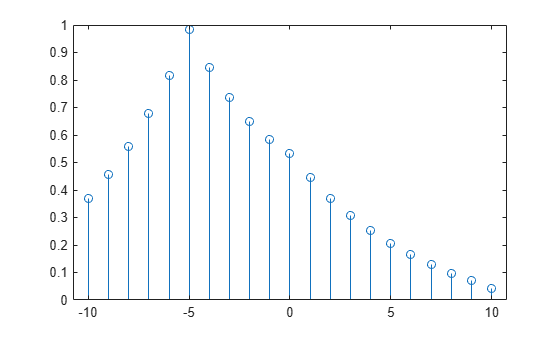# xcorr

Cross-correlation

## Syntax

``r = xcorr(x,y)``
``r = xcorr(x)``
``r = xcorr(___,maxlag)``
``r = xcorr(___,scaleopt)``
``````[r,lags] = xcorr(___)``````

## Description

example

````r = xcorr(x,y)` returns the cross-correlation of two discrete-time sequences. Cross-correlation measures the similarity between a vector `x` and shifted (lagged) copies of a vector `y` as a function of the lag. If `x` and `y` have different lengths, the function appends zeros to the end of the shorter vector so it has the same length as the other.```

example

````r = xcorr(x)` returns the autocorrelation sequence of `x`. If `x` is a matrix, then `r` is a matrix whose columns contain the autocorrelation and cross-correlation sequences for all combinations of the columns of `x`.```

example

````r = xcorr(___,maxlag)` limits the lag range from `-maxlag` to `maxlag` for either of the previous syntaxes.```

example

````r = xcorr(___,scaleopt)` also specifies a normalization option for the cross-correlation or autocorrelation. Any option other than `'none'` (the default) requires `x` and `y` to have the same length.```

example

``````[r,lags] = xcorr(___)``` also returns the lags at which the correlations are computed.```

## Examples

collapse all

Create a vector `x` and a vector `y` that is equal to `x` shifted by 5 elements to the right. Compute and plot the estimated cross-correlation of `x` and `y`. The largest spike occurs at the lag value when the elements of `x` and `y` match exactly (-5).

```n = 0:15; x = 0.84.^n; y = circshift(x,5); [c,lags] = xcorr(x,y); stem(lags,c)```Compute and plot the estimated autocorrelation of a vector `x`. The largest spike occurs at zero lag, when `x` matches itself exactly.

```n = 0:15; x = 0.84.^n; [c,lags] = xcorr(x); stem(lags,c)```Compute and plot the normalized cross-correlation of vectors `x` and `y` with unity peak, and specify a maximum lag of `10`.

```n = 0:15; x = 0.84.^n; y = circshift(x,5); [c,lags] = xcorr(x,y,10,'normalized'); stem(lags,c)```## Input Arguments

collapse all

Input array, specified as a vector, matrix, or multidimensional array. If `x` is a multidimensional array, then `xcorr` operates column-wise across all dimensions and returns each autocorrelation and cross-correlation as the columns of a matrix.

Data Types: `single` | `double`
Complex Number Support: Yes

Input array, specified as a vector.

Data Types: `single` | `double`
Complex Number Support: Yes

Maximum lag, specified as an integer scalar. If you specify `maxlag`, the returned cross-correlation sequence ranges from `-maxlag` to `maxlag`. If you do not specify `maxlag`, the lag range equals 2N – 1, where N is the greater of the lengths of `x` and `y`.

Data Types: `single` | `double`

Normalization option, specified as one of the following.

• `'none'` — Raw, unscaled cross-correlation. `'none'` is the only valid option when `x` and `y` have different lengths.

• `'biased'` — Biased estimate of the cross-correlation:

`${\stackrel{^}{R}}_{xy,\text{biased}}\left(m\right)=\frac{1}{N}{\stackrel{^}{R}}_{xy}\left(m\right).$`
• `'unbiased'` — Unbiased estimate of the cross-correlation:

`${\stackrel{^}{R}}_{xy,\text{unbiased}}\left(m\right)=\frac{1}{N-|m|}{\stackrel{^}{R}}_{xy}\left(m\right).$`
• `'normalized'` or `'coeff'` — Normalizes the sequence so that the autocorrelations at zero lag equal 1:

`${\stackrel{^}{R}}_{xy,\text{coeff}}\left(m\right)=\frac{1}{\sqrt{{\stackrel{^}{R}}_{xx}\left(0\right){\stackrel{^}{R}}_{yy}\left(0\right)}}{\stackrel{^}{R}}_{xy}\left(m\right).$`

## Output Arguments

collapse all

Cross-correlation or autocorrelation, returned as a vector or matrix.

If `x` is an M × N matrix, then `xcorr(x)` returns a (2M – 1) × N2 matrix with the autocorrelations and cross-correlations of the columns of `x`. If you specify `maxlag`, then `r` has size (2 × `maxlag` + 1) × N2.

For example, if `S` has three columns, $\text{S}=\left(\begin{array}{ccc}{x}_{1}& {x}_{2}& {x}_{3}\end{array}\right)$, then the result of `R = xcorr(S)` is organized as

`$\text{R}=\left(\begin{array}{lllllllll}{R}_{{x}_{1}{x}_{1}}\hfill & {R}_{{x}_{1}{x}_{2}}\hfill & {R}_{{x}_{1}{x}_{3}}\hfill & {R}_{{x}_{2}{x}_{1}}\hfill & {R}_{{x}_{2}{x}_{2}}\hfill & {R}_{{x}_{2}{x}_{3}}\hfill & {R}_{{x}_{3}{x}_{1}}\hfill & {R}_{{x}_{3}{x}_{2}}\hfill & {R}_{{x}_{3}{x}_{3}}\hfill \end{array}\right).$`

Lag indices, returned as a vector.

collapse all

### Cross-Correlation and Autocorrelation

The result of `xcorr` can be interpreted as an estimate of the correlation between two random sequences or as the deterministic correlation between two deterministic signals.

The true cross-correlation sequence of two jointly stationary random processes, xn and yn, is given by

`${R}_{xy}\left(m\right)=E\left\{{x}_{n+m}{y}_{n}^{*}\right\}=E\left\{{x}_{n}{y}_{n-m}^{*}\right\},$`

where  < n < , the asterisk denotes complex conjugation, and E is the expected value operator. `xcorr` can only estimate the sequence because, in practice, only a finite segment of one realization of the infinite-length random process is available.

By default, `xcorr` computes raw correlations with no normalization:

`${\stackrel{^}{R}}_{xy}\left(m\right)=\left\{\begin{array}{ll}\sum _{n=0}^{N-m-1}{x}_{n+m}{y}_{n}^{\ast },\hfill & m\ge 0,\hfill \\ {\stackrel{^}{R}}_{yx}^{*}\left(-m\right),\hfill & m<0.\hfill \end{array}$`

The output vector, `c`, has elements given by

`$\text{c}\left(\text{m}\right)={\stackrel{^}{R}}_{xy}\left(m-N\right),\text{ }\text{ }m=1,\text{\hspace{0.17em}}2,\dots ,2N-1.$`

In general, the correlation function requires normalization to produce an accurate estimate. You can control the normalization of the correlation by using the input argument `scaleopt`.

 Buck, John R., Michael M. Daniel, and Andrew C. Singer. Computer Explorations in Signals and Systems Using MATLAB®. 2nd Edition. Upper Saddle River, NJ: Prentice Hall, 2002.

 Stoica, Petre, and Randolph Moses. Spectral Analysis of Signals. Upper Saddle River, NJ: Prentice Hall, 2005.